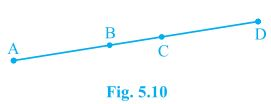Newbie

# In Fig. 5.10, if AC = BD, then prove that AB = CD. Q.6

• 0

How i solve the ncert class 9th question of chapter linear equations in two variables of exercise 4.3 question number 1(1). Please give me the simplest and easiest solution of this question  ,also give me the best solution of this question. In Fig. 5.10, if AC = BD, then prove that AB = CD

Share

1. Solution:It is given, AC = BD

From the given figure, we get,

AC = AB+BC

BD = BC+CD

⇒ AB+BC = BC+CD [AC = BD, given]

We know that, according to Euclid’s axiom, when equals are subtracted from equals, remainders are also equal.

Subtracting BC from the L.H.S and R.H.S of the equation AB+BC = BC+CD, we get,

AB+BC-BC = BC+CD-BC

AB = CD

Hence Proved.

• 0